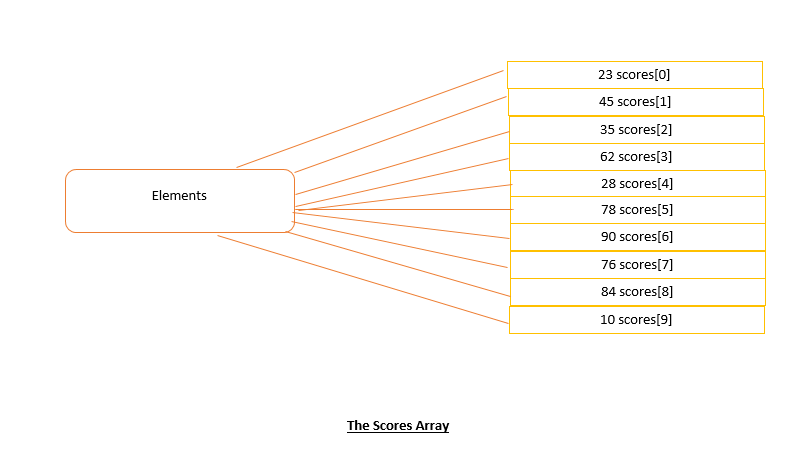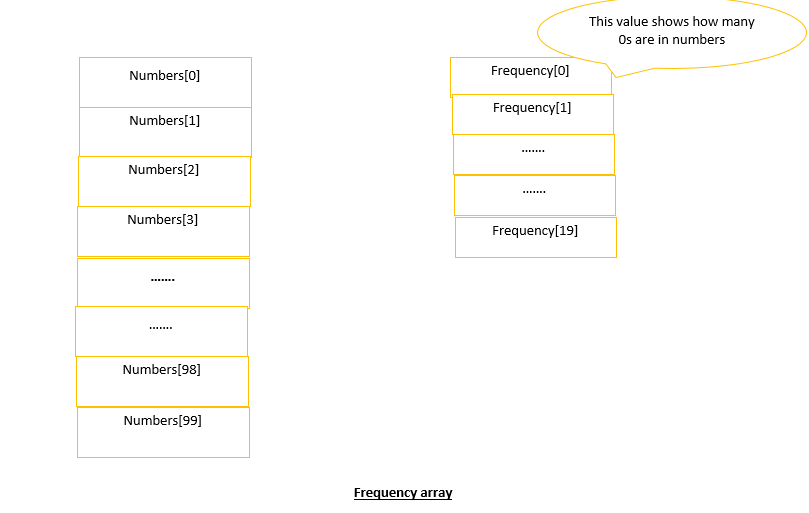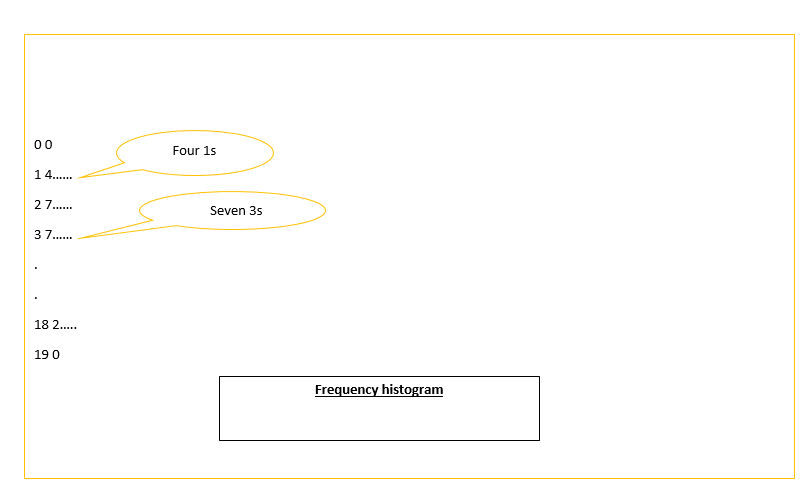# Arrays in C++ programming

By  Falak Hasija    97 - 03 August, 20Share article on social media

## What is an array?

An array is a sequenced collection of elements of the same data type.

Why do we need to study even when we can declare data types individually?

Suppose, we need 10 integers to be printed. We need 10 variables  to store 10 integers. Then to read them from the Keyboard we might need 10 read statements. This can be done but now think of a larger data, supposedly, 1000 or 100000000 integers to be stored........but now can we do this burdensome task??

This is where Arrays come to convert this tiresome task into an easy-going one!Using arrays in C++

Declaration and definition: Declaration and definition tell the compiler the name of the array, the type of data sotred in each element.

 int scores;

int is the datatype of each element contained in the array and scores is the name of the array, while the size of the array is 10 i.e. it can hold a maximum of 10 values.

Accessing elements in an array: C++ uses an index to access individual elements in an array. The index must be an integral value or an expression that evaluates to an integer. The array index in C++ starts from 0.

We could access the first element as follows:

 scores

However, in order to process all the elements at once, we must use a loop:

 for(i=0; i<10; i++) { scores[i]; }

Initializing arrays: Initializing all elements of the array can be done at the time of declaration and definition, just as with variables.

 int scores={ 20, 44, 50, 35, 60, 75, 83, 69, 10, 55 };

Inputting values: This can be done using a loop.

 for(i=0; i<10; i++) { cin>>scores[i]; }

Assigning values: Individual elements of an array can be assigned values using the assignment operator.

 scores  = 45;

To copy  an array to another array both of the same size, we can use a loop:

 for(i=0; i<20; i++) { holdscore[i] = scores[i]; }

If the values of an array follow the same pattern, we can use a loop to assign values.

 for(i=0; i<10; i++) { scores[i] = i*2; }

Exchanging values: A common application is to exchange the contents of two elements.

 scores = scores; scores = scores;

Outputting values: Another common application is to output the values.

 for(i=0; i<10; i++) { cout< }

Index range checking: The C++ language does not check the boundary of an array, so it is the programmer's job to ensure that all the references to indexed elements valid, and within the range of the array. This is called an index range checking.

Arrays and Functions:

To process arrays in a larger program, we have to able to pass them to functions. This can be done by:

1. Passing individual elements.

2. Passing the whole array.

Passing individual elements: Individual elements can be passed to a function like any ordinary variable.  As long as the array element type matches the function parameter type, it can be passed. Of course, it will be passed as a value parameter, which means that the function cannot change the value of the array element in the calling function.

Passing the whole array: If we want the function to operate on the whole array, we must pass the whole array.

1. In the calling function, you simply use the array name as the actual parameter.

2. In the calling function, you must declare the corresponding formal parameter in an array, the size of the array need not be specified.

Array Applications:

The following applications are:

1. Frequency Arrays: A frequency array shows the number of elements with an identical value found in a series of numbers. For example, Suppose we have taken a sample of 100 values between 0 and 19. We want to know how many times are the values are 0, how many are 1, and so on.

The way of doing this is to assign a value from the data array to an index and then use the index to access the frequency array.

 f = numbers[i]; frequency [f]++;2. Histograms: A histogram is a pictorial representation of a frequency array. Instead of printing the values of the elements to show the frequency of each number, we can print a histogram in the form of a bar chart.